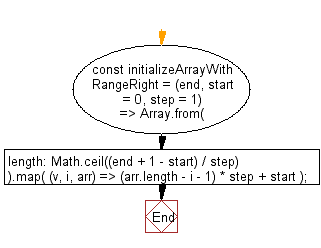# JavaScript: Initializes an array containing the numbers in the specified range (in reverse) where start and end are inclusive with their common difference step

## JavaScript fundamental (ES6 Syntax): Exercise-214 with Solution

Write a JavaScript program to initialize an array containing the numbers in the specified range (in reverse) where start and end are inclusive of their common difference step.

• Use Array.from(Math.ceil((end+1-start)/step)) to create an array of the desired length(the amounts of elements is equal to (end-start)/step or (end+1-start)/step for inclusive end), Array.prototype.map() to fill with the desired values in a range.
• Omit the second argument, start, to use a default value of 0.
• Omit the last argument, step, to use a default value of 1.

Sample Solution:

JavaScript Code:

``````//#Source https://bit.ly/2neWfJ2
const initializeArrayWithRangeRight = (end, start = 0, step = 1) =>
Array.from({ length: Math.ceil((end + 1 - start) / step) }).map(
(v, i, arr) => (arr.length - i - 1) * step + start
);

console.log(initializeArrayWithRangeRight(5));
console.log(initializeArrayWithRangeRight(7, 3));
console.log(initializeArrayWithRangeRight(9, 0, 2));
```
```

Sample Output:

```[5,4,3,2,1,0]
[7,6,5,4,3]
[8,6,4,2,0]
```

Pictorial Presentation:Flowchart:Live Demo:

See the Pen javascript-fundamental-exercise-214 by w3resource (@w3resource) on CodePen.

Improve this sample solution and post your code through Disqus

What is the difficulty level of this exercise?

Test your Programming skills with w3resource's quiz.

﻿